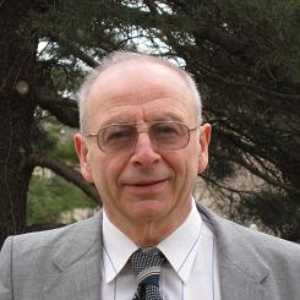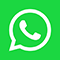HYBRID EVENT: You can participate in person at Paris, France or Virtually from your home or work.Kansas State University, United States

#### Abstract:

The Navier-Stokes  problem in R3  consists of solving the equations:

v0 + (v, ∇)v = −∇p + ν ?v + f,   x ∈ R3 ,  t ≥ 0,    ∇ · v = 0,    v(x, 0) = v0(x),

where v = v(x, t) is the  velocity of the  incompressible  viscous fluid, p = p(x, t) is the  pressure,  the  density  of the  fluid is ρ = 1, f = f (x, t) is the  exterior  force, v0 = v0(x) is the initial  velocity.

The  aim  of this  talk  is to  analyse  the  Navier-Stokes  problem  (NSP)  in  R3 without  boundaries. It  is proved  that the  NSP  is contradictory in the  following sense:

If  one  assumes that the initial data v(x, 0) 6≡ 0, ∇ · v(x, 0) = 0 and the solution to the NSP exists for  all  t ≥ 0, then one  proves that the solution v(x, t) to the NSP has  the property v(x, 0) = 0.

This  paradox  (the NSP paradox) shows that the  NSP  is not  a correct  de- scription  of the  fluid mechanics  problem  for incompressible  viscous fluid and  the NSP does not  have a solution  defined for all t > 0.  In the  exceptional  case, when the  data  are equal to zero, the  solution  v(x, t) to the  NSP does exist for all t ≥ 0 and is equal to zero, v(x, t) ≡ 0.

These results  are proved in –.

These results  solve one of the millennium  problems.

#### Biography:

Alexander G. Ramm is the author of 660 research papers and 13 monographs in analysis, applied mathematics, theoretical numerical analysis, ill-posed and inverse problems, and mathematical physics. He has given many lectures at various Universities throughout the world. He has several professional honors: Distinguished Visiting Professorships in UK, Mexico, Egypt, Mercator Professorship, Plenary talks at many Conferences, Khwarizmi International award. He is a member of editorial boards in many professional Journals. His main fields of expertise are: spectral and scattering theory, inverse and ill-posed problems, theoretical numerical mathematics, tomography, random fields estimation, wave scattering by many small particles and creating materials with a desired refraction coefficient.